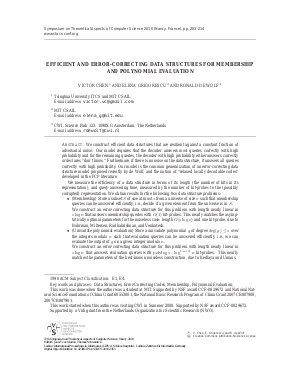Document# Efficient and Error-Correcting Data Structures for Membership and Polynomial Evaluation

### Authors Victor Chen, Elena Grigorescu, Ronald de Wolf## File

LIPIcs.STACS.2010.2455.pdf
• Filesize: 130 kB
• 12 pages

## Cite As

Victor Chen, Elena Grigorescu, and Ronald de Wolf. Efficient and Error-Correcting Data Structures for Membership and Polynomial Evaluation. In 27th International Symposium on Theoretical Aspects of Computer Science. Leibniz International Proceedings in Informatics (LIPIcs), Volume 5, pp. 203-214, Schloss Dagstuhl - Leibniz-Zentrum für Informatik (2010)
https://doi.org/10.4230/LIPIcs.STACS.2010.2455

## Abstract

We construct efficient data structures that are resilient against a constant fraction of adversarial noise. Our model requires that the decoder answers \emph{most} queries correctly with high probability and for the remaining queries, the decoder with high probability either answers correctly or declares don't know.'' Furthermore, if there is no noise on the data structure, it answers \emph{all} queries correctly with high probability. Our model is the common generalization of an error-correcting data structure model proposed recently by de~Wolf, and the notion of relaxed locally decodable codes'' developed in the PCP literature. We measure the efficiency of a data structure in terms of its \emph{length} (the number of bits in its representation), and query-answering time, measured by the number of \emph{bit-probes} to the (possibly corrupted) representation. We obtain results for the following two data structure problems: \begin{itemize} \item (Membership) Store a subset $S$ of size at most $s$ from a universe of size $n$ such that membership queries can be answered efficiently, i.e., decide if a given element from the universe is in $S$. \\ We construct an error-correcting data structure for this problem with length nearly linear in $s\log n$ that answers membership queries with $O(1)$ bit-probes. This nearly matches the asymptotically optimal parameters for the noiseless case: length $O(s\log n)$ and one bit-probe, due to Buhrman, Miltersen, Radhakrishnan, and Venkatesh. \item (Univariate polynomial evaluation) Store a univariate polynomial $g$ of degree $\deg(g)\leq s$ over the integers modulo $n$ such that evaluation queries can be answered efficiently, i.e., we can evaluate the output of $g$ on a given integer modulo $n$. \\ We construct an error-correcting data structure for this problem with length nearly linear in $s\log n$ that answers evaluation queries with $\polylog s\cdot\log^{1+o(1)}n$ bit-probes. This nearly matches the parameters of the best-known noiseless construction, due to Kedlaya and Umans. \end{itemize}
##### Keywords
• Data Structures
• Error-Correcting Codes
• Membership
• Polynomial Evaluation

## Metrics

• Access Statistics
• Total Accesses (updated on a weekly basis)
0